Get instant live expert help with Excel or Google Sheets“My Excelchat expert helped me in less than 20 minutes, saving me what would have been 5 hours of work!”

#### Post your problem and you’ll get Expert help in seconds.

Your message must be at least 40 characters
Our professional Expert are available now. Your privacy is guaranteed.

# Basic INDEX MATCH exact

While working with Excel, we are able to retrieve data from a dataset based on an exact match by using INDEX and MATCH functions.  The INDEX function returns a value as specified from within a range, while the MATCH function returns the position of a value in a range.

This step by step tutorial will assist all levels of Excel users in retrieving information based on an exact match.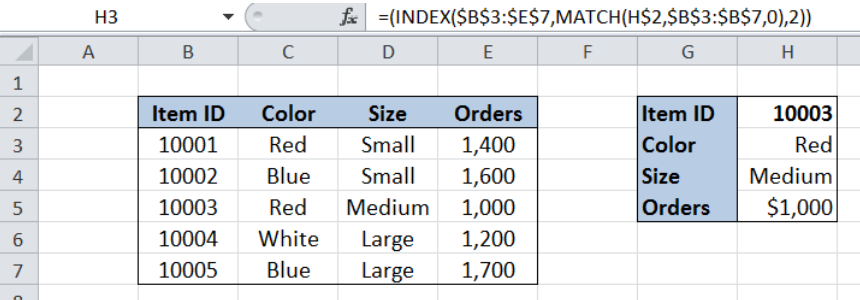Figure 1. Final result: Using Basic INDEX and exact MATCH

Final formula: `=(INDEX(\$B\$3:\$E\$7,MATCH(H\$2,\$B\$3:\$B\$7,0),2))`

## Syntax of the INDEX function

`=INDEX(array, row_num, column_num)`

The parameters are:

• array – a range of cells where we want to retrieve some data
• row_num – the row in the array from which we want to retrieve data
• column_num – the column in the array from which we want to retrieve data; if the array has only one column, column_num can be omitted

## Syntax of the MATCH function

`=MATCH(lookup_value, lookup_array, [match_type])`

The parameters are:

• lookup_value – a value which we want to find in the lookup_array
• lookup_array – the range of cells containing the value we want to match
• [match_type] optional; the type of match; if omitted, the default value is 1; We use 0 to find an exact match

## Setting up Our Data

Our table contains a list of Item ID (column B), Color (column C), Size (column D) and Orders (column E). In cell H2, we enter “10003”, which is the  Item ID that we want to match. In cells H3:H5, we want to retrieve the data for Color, Size and Orders of Item ID 10003.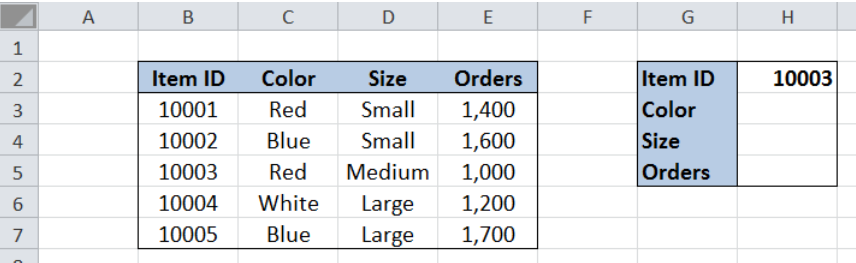Figure 2. Sample data to retrieve specific data based on exact match

## Retrieve data using INDEX and MATCH

In order to retrieve the data for Color, Size and Orders of Item ID 10003, we follow these steps:

Step 1.  Select cell H3

Step 2.  Enter the formula: `=(``INDEX(\$B\$3:\$E\$7,MATCH(H\$2,\$B\$3:\$B\$7,0),2))`

Step 3: Press ENTER

Step 4: Copy the formula in cell H3 to cells H4:H5 by clicking the “+” icon at the bottom right-corner of cell H3 and dragging it down

The dollar symbols “\$” in the formula fix the cells so that we can easily copy and paste the formula to other cells.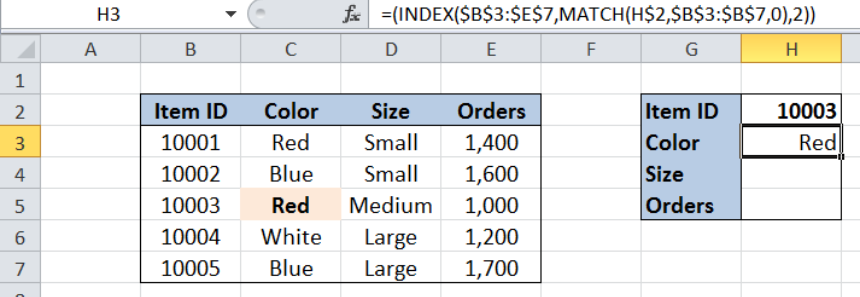Figure 3. Entering the formula to retrieve the matching color of item 10003

Our array for the INDEX function is the range B3:E7 which contains our data.  The row number is determined through the MATCH function, which finds the exact match and returns the position of cell H2, or Item ID “10003” in the range B3:B7.  The column number is 2, because the column “Color” is the second column in our array.

As a result, our formula returns “Red”in cell H3, which is the color of Item 10003.

Cells H4 and H5 return the corresponding size and orders for Item 10003, as shown below.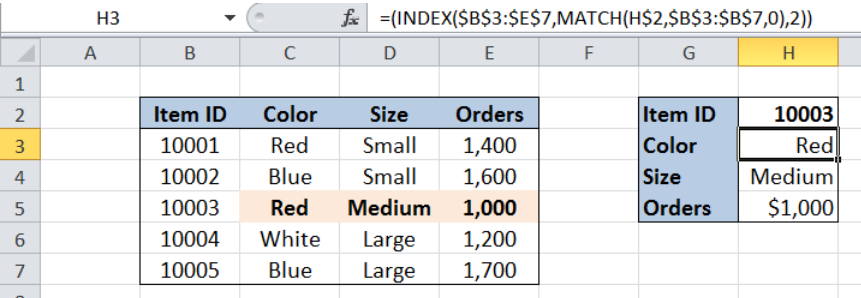Figure 4. Output: Using INDEX and MATCH to retrieve data based on exact match

## INDEX formula with only one column

It is also possible to work with the INDEX formula using only one column.  We can rewrite the formula and use the needed range as the array, so that there is no need to supply the INDEX formula with a column number.

In column C11 below, we want to retrieve the data for color of Item 10003.  In C11, we enter the formula =(INDEX(C3:C7,MATCH(C\$9,\$B\$3:\$B\$7,0))).  Note that the array used “C3:C7” is only in column C.

The result is “Red”, which is the same as in the previous example.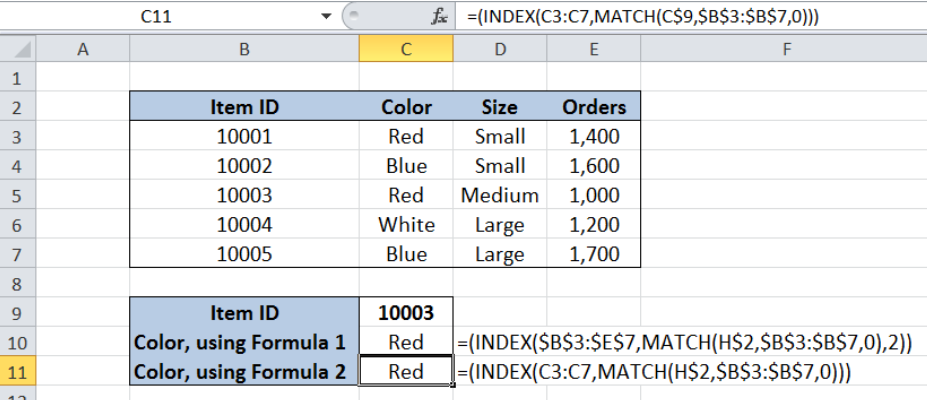Figure 5. Comparison: INDEX formula using multiple or single column

Most of the time, the problem you will need to solve will be more complex than a simple application of a formula or function. If you want to save hours of research and frustration, try our live Excelchat service! Our Excel Experts are available 24/7 to answer any Excel question you may have. We guarantee a connection within 30 seconds and a customized solution within 20 minutes.

### Did this post not answer your question? Get a solution from connecting with the expert.Another blog reader asked this question today on Excelchat:
Solution examplesindex and match with duplicates. I need to use another column as a reference, so my return value has two match the value of two things for it to return
Solved by E. H. in 60 minsHello, I have a big spreadsheet in which I need to know how many patients came in each month based on provider. I am using the following formula but I still get the N/A error. =INDEX('No Show Appts Data'!D:E, MATCH(1, 'No Show Appts Data'!D:D='No Shows Data'!M2)*('No Shows Data'!E:E='No Shows Data'!N1),0)) No Show Appts Data is the name of the sheet where Column D is Month Year of date in question and Column E is the provider. No Shows Data is the sheet where I am making all the formula calculation where Column M is Month and Year and Column N is the provider in question and therefore M2 is the month in question and N1 the provider in question. How do I fix this error? Thanks
Solved by F. H. in 40 minsI need to find an INDEX function that will convert the Call Day (a number 1-7) to the actual weekday found in row 1 of the DayofWeek named range. I don't know if I'm supposed to use the MATCH function as well or not.
Solved by D. D. in 11 minsI have two columns with names and a third one with email addresses. For every name that matches, I need to copy the email address to an empty column next to the matching name. .
Solved by E. U. in 15 minshelp with INDEX(IndexArea,MATCH(\$M\$5,MatchRows,0),MATCH(\$M\$6,MatchColumns,0))
Solved by Z. Y. in 12 mins## Subscribe to Excelchat.coAnother blog reader asked this question today on Excelchat: